# Trigonometric equations

## Summary and examples

Trigonometric equations are equations that contain one or more trigonometric functions. We will restrict ourselves to a few common trigonometric equations.
In solving such equations a (graphical) calculator is often - but not always -necessary. We try to limit the use of such calculators to a minimum, which increases understanding.
For example, let us look at the equation: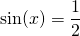Suppose we want to solve this equation with a calculator. We can find theas follows: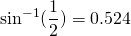Here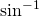is the inverse function of the function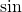. The latter function gives the value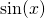for a certain value of, the inverse function does the inverse. If we give the value ofwe get the value ofin return. We can check this: when we want to calculate the inverse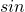function of the value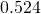(i.e.) we get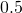(not exactly becauseis an approximation of).

We could have solved this equation without a calculator. The table with trigonometric values shows thatcorresponds to the value: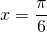We are not finished yet. Looking at the unit circle (see also Unit circle and simple formulas), we find that within the circle there is another value offor which thefunction gives the value. The first value lies in the first quadrant, but in the second quadrant there is another solution, namely: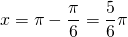This value ofis a solution of the equation as well. Actually, we know that also the following values ofare solutions: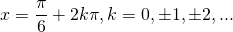because the period of the-function is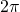.

Thus we have: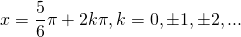In general the following holds.

If: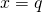is the solution of the equation that is found using a table or calculator:then the solutions of this equation are: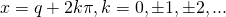or: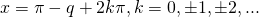In a similar way we solve the equation: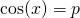If:is the solution of the equation that is found using a table or calculator:then the solutions of this equation are:or: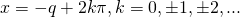Let us look at equations having the form: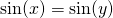or: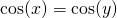These equations are solved in a similar way as shown above. The first type of these equations have the following solutions: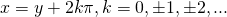or: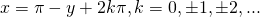For the second type of the equations we have:or: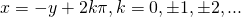There are also equations of the type: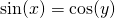In this case we may use the following formulas which can be deduced straightforward from the unit circle (see also Unit circle and simple formulas):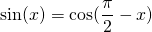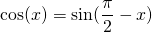##### Example 1

Solve: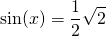The table shows: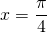Then we also know that a solution is: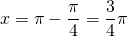Because the-function has the period, the solutions of the equation are: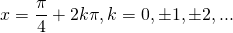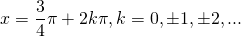##### Example 2

Solve: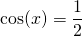The table shows: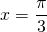Because the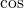-function has the period, the solutions of the equation are: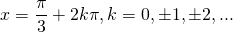or: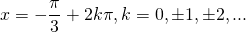##### Example 3

Solve: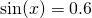In this case the value cannot be taken from a table and thus we need to use a calculator.

We find (approximated):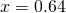and thus (also approximated):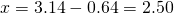Because the-function has the period, the solutions of the equation are (approximated):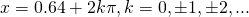##### Example 4

Solve: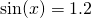In this case table nor calculator can help us, because the-function lies in the interval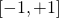(the so-called range) and therefore this equation does not have a solution.

##### Example 5

Solve: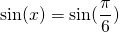The solution is straightforward: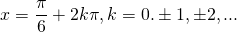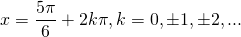0